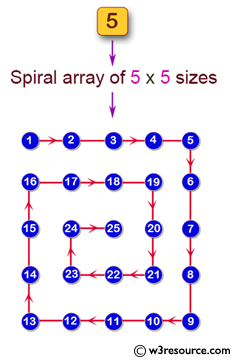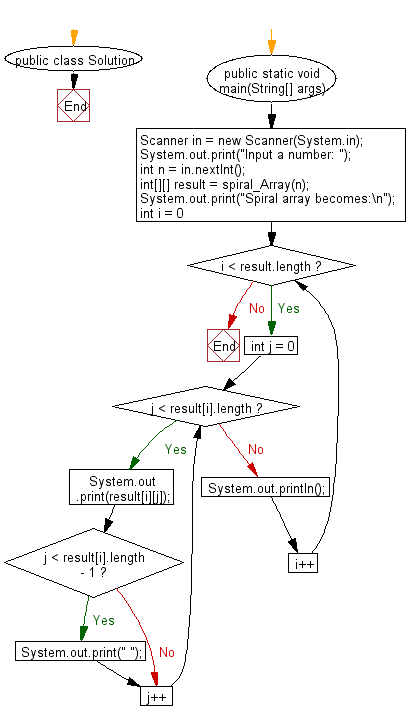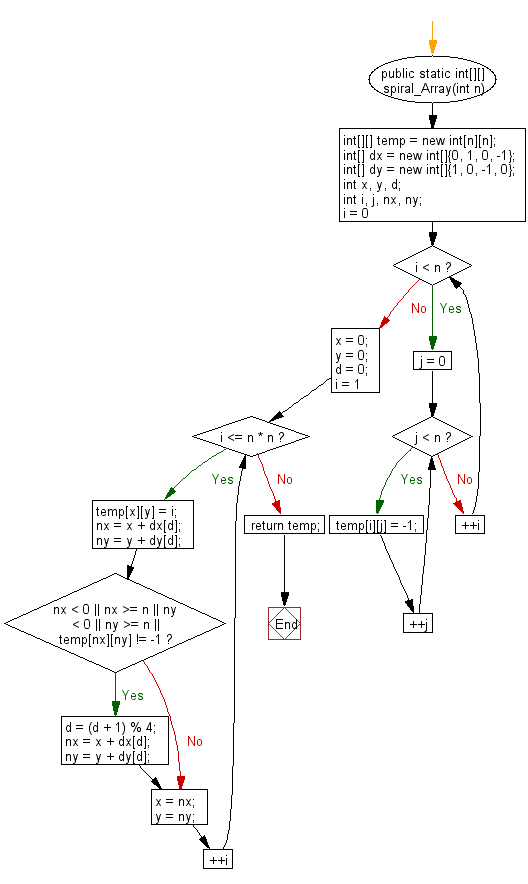# Java Exercises: Create a spiral array of n * n sizes from a given integer n

## Java Basic: Exercise-196 with Solution

Write a Java program to create a spiral array of n * n sizes from a given integer n.

Input number: 3
Output:
1 2 3
8 9 4
7 6 5

Pictorial Presentation:Sample Solution:

Java Code:

``````import java.util.*;
public class Solution {
public static void main(String[] args) {
Scanner in = new Scanner(System.in);
System.out.print("Input a number: ");
int n = in.nextInt();
int[][] result = spiral_Array(n);
System.out.print("Spiral array becomes:\n");
for(int i = 0; i < result.length; i++)
{
for(int j = 0; j < result[i].length; j++)
{
System.out.print(result[i][j]);
if(j < result[i].length - 1) System.out.print(" ");
}
System.out.println();
}
}
public static int[][] spiral_Array(int n) {
int[][] temp = new int[n][n];
int[] dx = new int[]{0, 1, 0, -1};
int[] dy = new int[]{1, 0, -1, 0};
int x, y, d;
int i, j, nx, ny;
for (i = 0; i < n; ++i)
{
for (j = 0; j < n; ++j)
{
temp[i][j] = -1;
}
}
x = 0;
y = 0;
d = 0;
for (i = 1; i <= n * n; ++i)
{
temp[x][y] = i;
nx = x + dx[d];
ny = y + dy[d];
if (nx < 0 || nx >= n || ny < 0 || ny >= n || temp[nx][ny] != -1) {
d = (d + 1) % 4;
nx = x + dx[d];
ny = y + dy[d];
}
x = nx;
y = ny;
}
return temp;
}
}
```
```

Sample Output:

```Input a number:  5
Spiral array becomes:
1 2 3 4 5
16 17 18 19 6
15 24 25 20 7
14 23 22 21 8
13 12 11 10 9
```

Flowchart:Java Code Editor:

What is the difficulty level of this exercise?

﻿

## Java: Tips of the Day

Count the number of occurrences of a char in a String:

```int count = StringUtils.countMatches("a.b.c.d", ".");
```

Ref: https://bit.ly/2OTy6sG